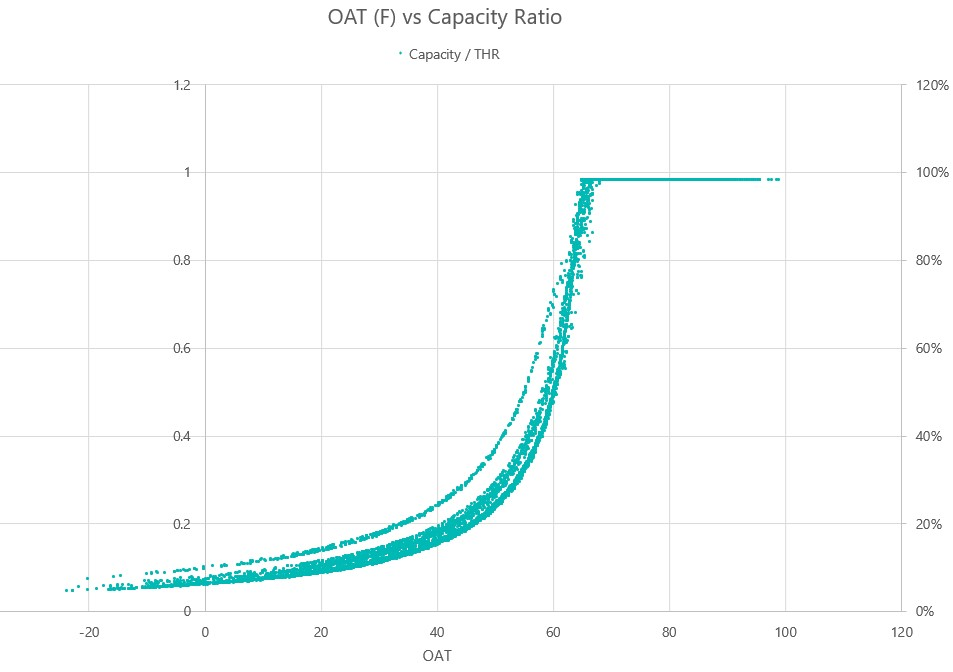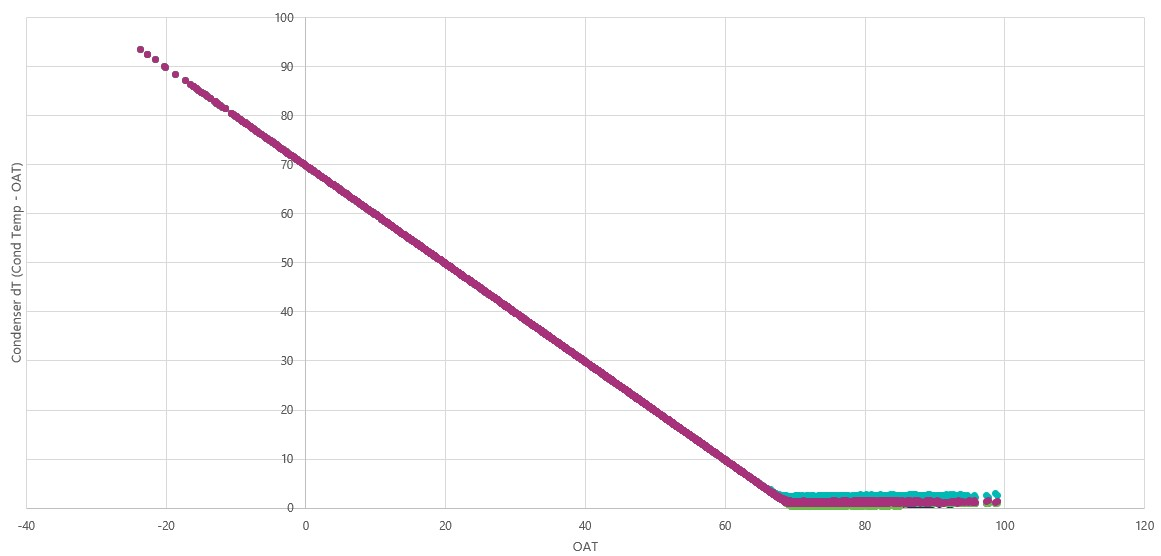Question-and-Answer Resource for the Building Energy Modeling Community
Get started with the Help page

# Refrigeration Air Cooled Condenser Curves - EnergyPlus

After spending some time with a grocery store model, it seems there is an issue with how the Refrigeration:Condenser:AirCooled object is controlling the condenser fans. This object has very few inputs to determine power and capacity, just the rated fan power and a linear curve for condenser capacity. If it is cold outside, everything operates as it should, the fan speed drops and the condensing temperature is set constant at the minimum specified in the input. When the outdoor air temperature rises above that minimum condensing temperature, the program forces the fans to run at 100% all the time. Instead these fans should operate to maintain a dT between the outdoor air temperature and condensing temperature.

For example, if it is 90F outside, the condensing temperature should be 100F if we are maintaining a 10F dT. instead, I am seeing the dT at 3-5F because the fans are running flat out, using way more energy than they should. This isn't a big deal for colder climates, but when I move this model to Miami, the condenser fans run at essentially 100% all year.

Looking at the [Engineering Reference], the condensing temperature is determined by a variable called Hrej divided by HrejRated rather than a constant dT when the outside air temp is warm. The system then thinks the condenser fans need to run at 100% to maintain this low condensing temperature.

So far, I have experimented with the linear curve coefficient, min/max values, speed control type, and minimum fan speed. Please let me know if I am missing something.

Here is the % capacity by outside air temperature:and the dT between outside air temp and condensing temperature:edit retag close merge delete

Sort by » oldest newest most voted

I dug a bit into the source code, and found some things that might help out here.

First, this statement:

the condensing temperature is determined by a variable called Hrej divided by HrejRated rather than a constant dT when the outside air temp is warm.

isn't quite correct. the condensing temperature is calculated by

    } else { // air-cooled condenser
// MinCondLoad and TempSlope came from condenser capacity curve, using curve backwards
TCondCalc = OutDbTemp + (state.dataRefrigCase->TotalCondenserHeat - condenser.MinCondLoad) * condenser.TempSlope;
SinkTemp = OutDbTemp;


i.e. OAT plus total condenser heat from compressor rack minus Minimum condenser load (determined from the condenser curve), multiplied by the inverse slope of the condenser curve (i.e. (x_max - x_min) / (Cap_max - Cap_min)).

Next, this statement:

When the outdoor air temperature rises above that minimum condensing temperature, the program forces the fans to run at 100% all the time.

is also not quite right. The fan operation is determined by comparing the calculated condenser temp (from above) with the input Minimum Condensing Temperature from the Refrigeration:System:

    // Fan energy calculations apply to both air- and evap-cooled condensers
// Compare calculated condensing temps to minimum allowed to determine fan power/operating mode
if (TCondCalc >= this->TCondenseMin) {
this->TCondense = TCondCalc;
ActualFanPower = RatedFanPower;
AirVolRatio = 1.0;

} else { // need to reduce fan speed to reduce air flow and keep Tcond at or above Tcond min
this->TCondense = this->TCondenseMin;
TCondCalc = this->TCondenseMin;
// recalculate CapFac at current delta T
if (condenser.CondenserType == DataHeatBalance::RefrigCondenserType::Air) {
// current maximum condenser capacity at delta T present for minimum condensing temperature [W]
Real64 CurMaxCapacity = CurveManager::CurveValue(state, condenser.CapCurvePtr, (this->TCondenseMin - OutDbTemp));
CapFac = state.dataRefrigCase->TotalCondenserHeat / CurMaxCapacity;
AirVolRatio = max(FanMinAirFlowRatio, std::pow(CapFac, CondAirVolExponentDry)); // Fans limited by minimum air flow ratio
AirVolRatio = min(AirVolRatio, 1.0);


Essentially, if the calculated condensing temp is >= the minimum, fans are on at 100%, else they're reduced to some fraction to maintain the minimum condensing temp.

So basically I don't think the condenser model can be controlled to a specific delta-T, because the condensing temperature is calculated based on the compressor load, and because the condenser curve is what it is. However, I think you could still resolve the high fan power issue, because 1. The Minimum Condensing Temperature input value is available as an EMS Actuator, and 2. (at least for air-cooled condensers) it only really seems to be used to determine the fan operation. So you could write an EMS program to calculate the condensing temp and reset the minimum condensing temp to be above that, up to maybe the design DT of the condenser at design OA. Or something to that effect.

I also thought about trying to actuate the output of the Rated Effective Total Heat Rejection Rate Curve, but I don't thing that's going to work. The curve is evaluated to determine the min/max capacities to invert the slope (as above) to calculate the condensing temperature. Since I think an EMS curve output actuator would override these min/max checks, it would mess with that whole process.

Hope this ...

more

Eric, thanks for the detailed analysis, this is really great stuff. We should be able to figure out the EMS to change the condensing temperature. Two questions for you, though: it looks like the compressor performance curve calculations are dependent on condensing temperature also, I assume the new condensing temperature will be picked up in the compressor curve, correct? Second, how do you find the source code for the EnergyPlus calculations?

1

Yeah it does look like the compressor curves are evaluated using TCondense, which will be TCondenseMin if the calculated temp is >= TCondenseMin. All the Refrigeration system code is in https://github.com/NREL/EnergyPlus/bl..., which is too big to render in Github (and why I didn't link to the actual lines). The CalculateCondensers routine starts at line 11876, and CalculateCondensers at 12471.